## 建模并验证系统

### 打开系统布局

```open_system('system_layout.slx') ```### 对组件进行建模

• 物理组件的输出和输入之间的显式数学关系 - 您可以根据组件的输入通过代数计算和微分方程积分，直接或间接计算出组件的输出。例如，按照给定的进水速率计算水箱中的水位就是一种显式关系。每个 Simulink 模块基于从输入到输出的计算定义来执行。

• 物理组件的模型变量之间的隐式数学关系 - 由于变量之间相互依赖，因此为组件指定输入和输出并不容易。例如，电路中连接的电机的 `+` 极电压和 `-` 极电压之间就存在一种隐式关系。要在 Simulink 中对这种关系进行建模，您可以使用 Simscape™ 等物理建模工具，也可以将这些变量建模为允许定义输入/输出的更大组件的一部分。有时候，更仔细地审视建模目的和组件定义有助于定义输入/输出关系。

• 从实际系统获得的数据 - 您已经测得实际组件的输入/输出数据，但不存在完全定义的数学关系。许多设备具有符合此描述的未建模组件。例如，电视机散发的热量。您可以使用 System Identification Toolbox™ 来定义此类系统的输入/输出关系。

• 显式功能定义 - 您可以根据功能组件的输入通过代数计算和逻辑计算来定义功能组件的输出。例如，调温器的开关逻辑。您可以将大多数功能关系建模为 Simulink 模块和子系统。

3. 分别为每个组件构建模型。构建系统模型最有效的方法是首先独立地考虑各个组件。

4. 首先，使用接近于系统的模型构建简单的模型。找出可能会影响模型准确性的假设条件。以迭代方式添加细节，直到复杂度满足建模和准确性要求。

#### 对物理组件进行建模

• 每个组件的常量是什么？什么值不会更改，除非您更改它们？

• 每个组件的变量是什么？什么值会随着时间而更改？

• 一个组件有多少个状态变量？

• 对于连续系统，微分方程描述变量的变化率，为所有时间值都定义方程。例如，一阶微分方程给出车速：

`$\frac{dv\left(t\right)}{dt}=-\frac{b}{m}v\left(t\right)+u\left(t\right)$`
• 对于离散系统，差分方程描述变量的变化率，但只在特定时间定义方程。例如，来自离散比例微分控制器的控制信号：

`$pd\left[n\right]=\left(e\left[n\right]-e\left[n-1\right]\right){K}_{d}+e\left[n\right]{K}_{p}$`
• 没有导数的方程是代数方程。例如，用代数方程表示包含两个组件的并联电路中的总电流：

`${I}_{t}={I}_{a}+{I}_{b}$`

• 电机施加的力 - 此力 F 作用在速度变化的方向上，是轮子子系统的输入。

• 阻力 - 此力 Fdrag 作用在速度变化的相反方向上，是速度的函数。

`${F}_{drag}={k}_{drag}V|V|$`

`$\begin{array}{c}\left(m/2\right)\stackrel{˙}{V}=F-{F}_{drag}\\ \left(m/2\right)\stackrel{˙}{V}=F-{k}_{drag}V|V|\\ \stackrel{˙}{V}=\frac{F-{k}_{drag}V|V|}{\left(m/2\right)}\end{array}$`

1. `system_layout` 模型中，双击 Right Wheel 子系统以显示空子系统。

2. 对速度和加速度进行建模。添加一个 Integrator 模块。将初始条件设置保留为 `0`。此模块的输入是加速度 Vdot，输出是速度 V。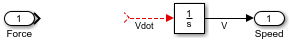3. 对阻力进行建模。从 User-Defined Functions 库中添加一个 MATLAB Function 模块。MATLAB Function 模块提供一种在模型中实现数学表达式的快速方法。要编辑函数，请双击该模块以打开 MATLAB Function 编辑器。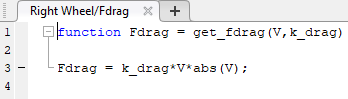4. 定义 MATLAB Function 模块的参数。在 MATLAB Function 模块编辑器中，点击编辑数据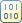按钮。点击 k_drag，将作用域设置为参数并点击应用

5. Subtract 模块从电机的作用力中减去阻力。使用带有参数 `1/(m/2)`Gain 模块完成力-加速度方程。

6. 要反转 MATLAB Function 模块的方向，请选择该模块。在工具条的格式选项卡上，点击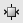。连接这些模块。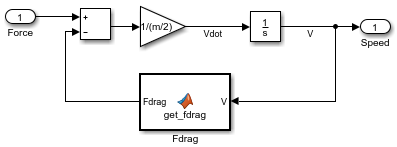7. 这两个轮子的动态特性相同。复制您刚刚建模的 Right Wheel 子系统，并将其粘贴到 Left Wheel 子系统中。

8. 查看模型的顶层。点击按钮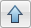`$\stackrel{˙}{\theta }=\frac{{V}_{R}-{V}_{L}}{2r}$`

1. `system_layout` 模型的顶层，双击 Rotation 子系统以显示空子系统。删除 InportOutport 模块之间的连接。

2. 对角速度和角度进行建模。添加一个 Integrator 模块。将初始条件设置保留为 `0`。此模块的输出是角度 theta，输入是角速度 theta_dot。

3. 根据切向速度计算角速度。添加带有参数 `1/(2*r)`Gain 模块。

4. 连接这些模块。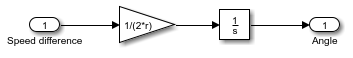5. 查看模型的顶层。点击按钮#### 对功能组件进行建模

`$\begin{array}{l}{V}_{X}={V}_{N}\mathrm{cos}\left(\theta \right)\\ {V}_{Y}={V}_{N}\mathrm{sin}\left(\theta \right)\end{array}$`

1. `system_layout` 模型的顶层，双击 Coordinate Transform 子系统以显示空子系统。

2. 对三角函数进行建模。从 Math Operations 库中添加一个 SinCos 模块。

3. 对乘法进行建模。从 Math Operations 库中添加两个 Product 模块。

4. 连接这些模块。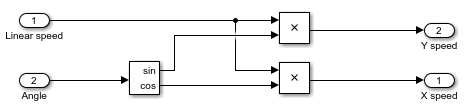5. 查看模型的顶层。点击按钮#### 设置模型参数

• 书面规范，如标准属性表或制造商的数据表

• 直接测量现有系统所得的测量值

• 基于系统输入/输出的估计值

Simulink 使用 MATLAB® 工作区来计算参数。可在 MATLAB 命令行窗口中设置这些参数：

```m = 2.5; k_drag = 30; r = 0.15;```

### 通过仿真来验证组件

• 当向轮子连续施加力时，速度会增加，直到达到稳定状态的速度为止。

• 当两个轮子向相反方向转动时，旋转角度以恒定速率增加。

#### 验证轮子组件

1. 创建一个新模型。在仿真选项卡中，点击新建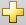。将 Right Wheel 模块复制到新模型中。

2. 创建一个测试输入。从 Sources 库中添加一个 Step 模块，并将其连接到 Right Wheel 模块的输入端口。将步长时间参数设置保留为 `1`

3. 在输出端口添加一个查看器。右键点击 Right Wheel 模块的输出端口，然后选择创建并连接查看器 > Simulink > Scope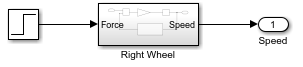4. 运行仿真。在仿真选项卡中，点击运行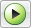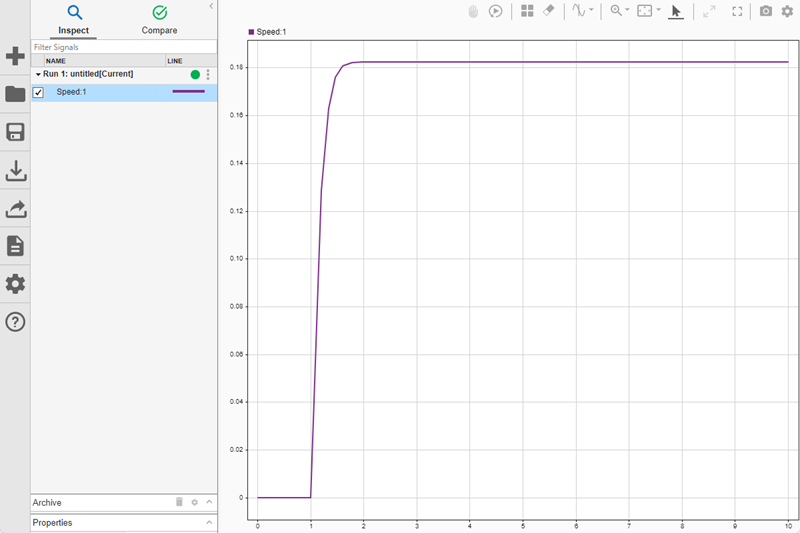#### 验证旋转组件

1. 创建一个新模型。点击，将 Rotation 模块复制到新模型中。

2. 在新模型中创建测试输入。从 Sources 库中添加一个 Step 模块。将步长时间参数设置保留为 `1`。将其连接到 Rotation 模块的输入端口。此输入表示当两个轮子沿相反方向旋转时的轮子速度之差。

3. 在输出端口添加一个查看器。右键点击 Rotation 模块的输出端口，然后选择创建并连接查看器 > Simulink > Scope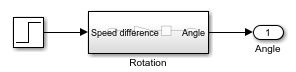4. 运行仿真。在仿真选项卡中，点击运行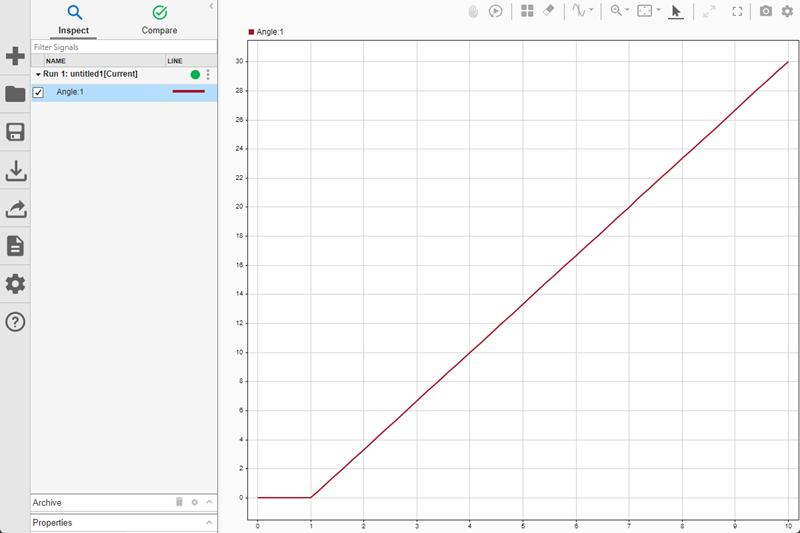• 您可以将弧度输出转换为角度。添加增益为 `180/pi`Gain 模块。

• 您可以按 360 度为一圈显示角度输出。添加带有函数 `mod`Math Function 模块。

MATLAB 三角函数采用弧度输入。

### 验证模型

• 当沿相同方向对两个轮子施加相同的力时，机器人沿直线运动。

• 当沿相反方向对两个轮子施加相同的力时，机器人原地旋转。

1. `system_layout` 模型中，双击 Inputs 子系统以显示空子系统。

2. 通过添加 Step 模块创建测试输入。将步长时间参数设置保留为 `1`。将其连接到两个 Outport 模块上。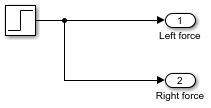3. 在模型的顶层，将两个输出信号连接到同一个波形查看器：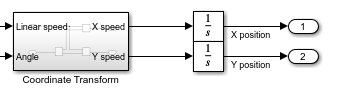4. 运行模型。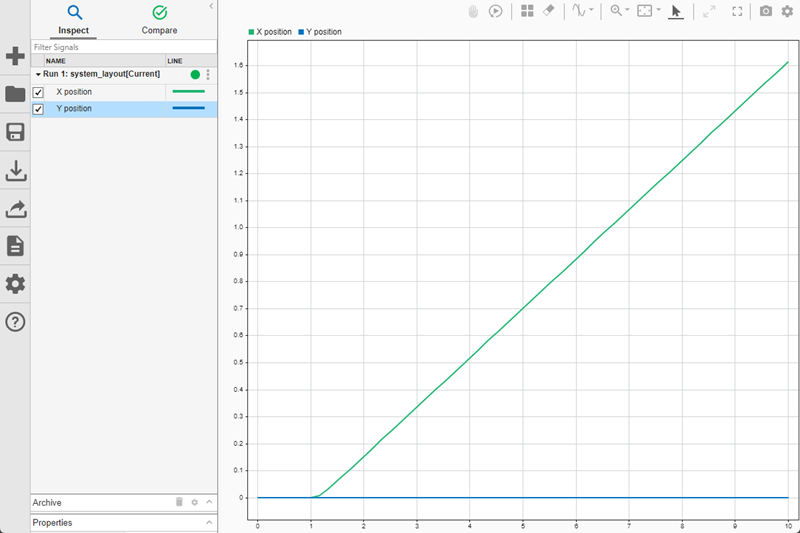在上图中，黄线是 X 方向，蓝线是 Y 方向。由于角度为零并且保持不变，因此机器人只在 X 方向上移动，跟预期一样。

5. 双击 Inputs 子系统，在信源和第二个输出之间添加具有参数 `-1`Gain 模块。这将反转左轮的方向。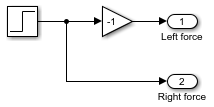6. 为角度输出添加一个示波器。

7. 运行模型。第一个视图显示 X-Y 平面上没有运动。第二个视图显示有稳定旋转。

• 当初始角度非零时会发生什么？

• 当作用力下降到零时，运动需要多长时间才能停止？

• 当机器人更重时会发生什么？

• 当机器人在更光滑的平面上移动时（即阻力系数更低时）会发生什么？